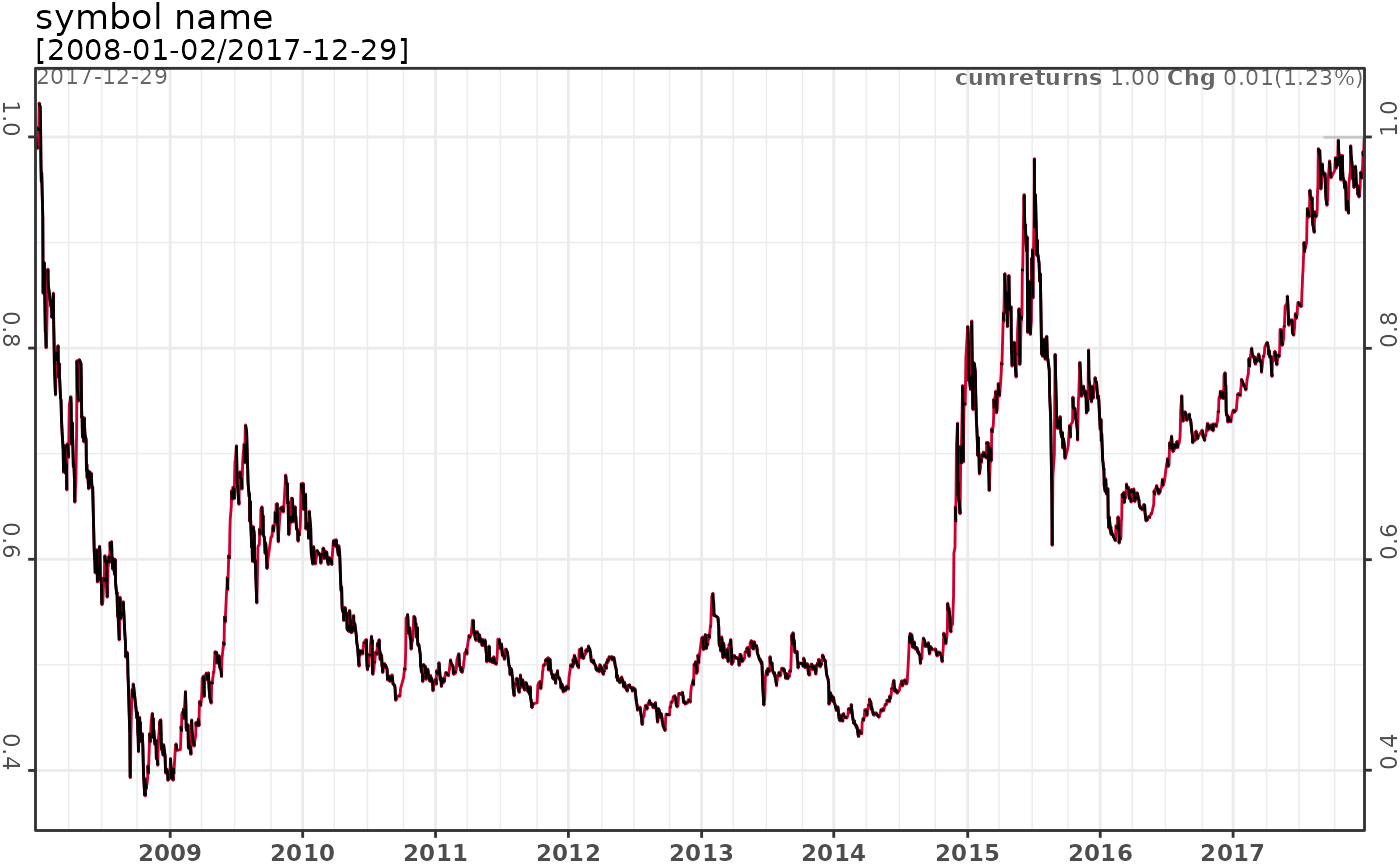pq_portfolio calculates the weight returns or the wealth index of a portfolio of assets.

pq_portfolio(dt, w = NULL, rcol = "returns", wcol = "weights",
wealthindex = FALSE, method = "arithmetic")

Arguments

dt a list/dataframe of returns by asset a dataframe of weights by asset. If it is NULL, then the weights by asset are equal. the returns column name the weights column name logical, whether to return a wealth index. Defaults to FALSE. the method to calculate asset returns, the available values include arithmetic and log, defaults to arithmetic.

Examples

data(dt_banks)